# BITSAT Mathematics Application Of Integrals MCQs

BITSAT Mathematics Application Of Integrals MCQs with answers available in Pdf for free download. The MCQ Questions for BITSAT Mathematics with answers have been prepared as per the latest BITSAT Mathematics syllabus, books and examination pattern. Multiple Choice Questions form important part of competitive exams and BITSAT exam and if practiced properly can help you to get higher rank. Refer to more topic wise BITSAT Mathematics Questions and also download more latest study material for all subjects and do free BITSAT Mathematics Mock Test

## MCQ for BITSAT Mathematics Application Of Integrals

BITSAT Mathematics students should refer to the following multiple-choice questions with answers for Application Of Integrals in BITSAT. These MCQ questions with answers for BITSAT Mathematics will come in exams and help you to score good marks

### Application Of Integrals MCQ Questions with Answers

• a) 2 √2
• b) 2 √2 - 2
• c) 2 √2 + 2
• d) 0

#### Question: The area of the region R =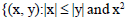is

• a)• b)• c)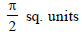• d)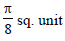#### Question: The area bounded by the x-axis, the curve y = f(x) and the lines x =1, x =b, is equal to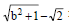for all b > 1, then f(x) is

• a)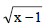• b)• c)• d)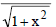#### Question: Area intercepted by the curves y = cos x, x €[0, π] and y = cos 2x, x Î[0,π] , is

• a)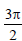• b)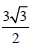• c)• d)• a) π
• b) π/2
• c) 1
• d) 2

#### Question: What is the area bounded by y = tan x , y = 0 and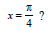• a) ln 2 sq. units
• b)• c) 2 (ln 2) sq. units
• d) None of these#### Question: The area of the region bounded by the curve y = x | x |, x-axis and the ordinates x = 1, x = -1is given by :

• a) zero
• b)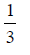• c)• d) 1#### Question: Area of the triangle formed by the line x + y = 3 and angle bisectors of the pair of straight lines x2 – y2 + 2y =1 is

• a) 2 sq. units
• b) 4 sq. units
• c) 6 sq. units
• d) 8 sq. units

#### Question: The area between the parabola y = x2 and the line y = x is:

• a)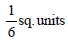• b)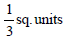• c)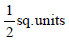• d)

None of these#### Question: The area between the curve y = 1 – |x| and the x – axis is equal to

• a) 1 sq. unit
• b)• c)• d) 2 sq. units

#### Question: The area of the figure bounded by y = ex, y = ex and x = 1 is

• a) 2(e – 1)
• b)• c)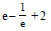• d)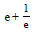• a) 2
• b) 1
• c) 1/2
• d) 1/4

#### Question: The area bounded by the x-axis, the curve y = f(x) and the lines x =1, x =b, is equal tofor all b > 1, then f(x) is

• a)• b)• c)• d)#### Question: The area of the region R =is

• a)• b)• c)• d)• a) 2 √2
• b) 2 √2 - 2
• c) 2 √2 + 2
• d) 0